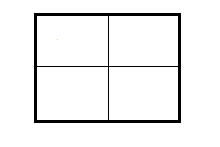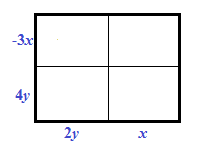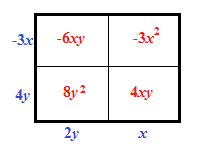### Home > CCA > Chapter 11 > Lesson 11.1.1 > Problem11-14

11-14.

Multiple Choice: Which of the following expressions is the product of $\left(4y-3x\right)\left(2y+x\right)$?

1. $8y^2-2xy-3x^2$

1. $6y^2-2xy-2x^2$

1. $8y^2+10xy-3x^2$

1. $6y^2-2x$

Use a generic rectangle to multiply the expression together. Draw a rectangle and divide it into four equal parts.

Multiply each row by each column. Add the products together to get the final product. Added to the rectangle: Exterior left edge top is negative 3, X. Exterior left edge bottom is 4, y. Exterior bottom edge left is 2, y. Exterior bottom edge right is x.

$(4y−3x)(2y+x)=8y^2−2xy−3x^2$ Added to the rectangle: Interior upper left box is negative 6, x, y. Interior upper right box is negative 3, X squared. Interior lower left box is negative 8, y squared. Interior lower right box is 4, X, Y.